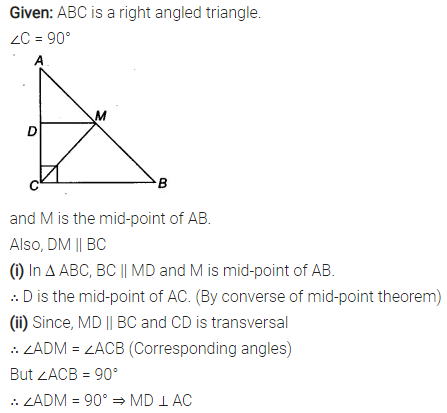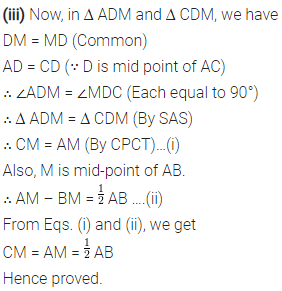# ABC is a triangle right angled at C. A line through the mid-point M

ABC is a triangle right angled at C. A line through the mid-point M of hypotenuse AB and parallel to BC intersects AC at D. Show that
(i) D is the mid-point of AC
(ii) MD ⊥ AC
(iii) CM = MA = \frac { 1 }{ 2 } AB